Follow

# How to Setup Equal Interval Grading

In this article, we discuss several ways you can implement the “Equal Interval” methodology using the QuickSchools Gradebook.

## Introduction

The Equal Interval philosophy has been very controversial to say the least. When assessing a student's performance over time (presumably in your Gradebook), how would you weigh an F vs. an A? If a student received an F in one assignment, and an A in the next assignment, what should the average be?

Traditionally, if a student receives an F and an A and you simply sought an average Final Grade, you’d average the marks. But an A typically covers the top 10%, while an F covers the bottom 60%. And so averaging a 100%-A and a 0%-F gets you an F at 50%. And the effort put in by the student who received an A is lost in the final grade.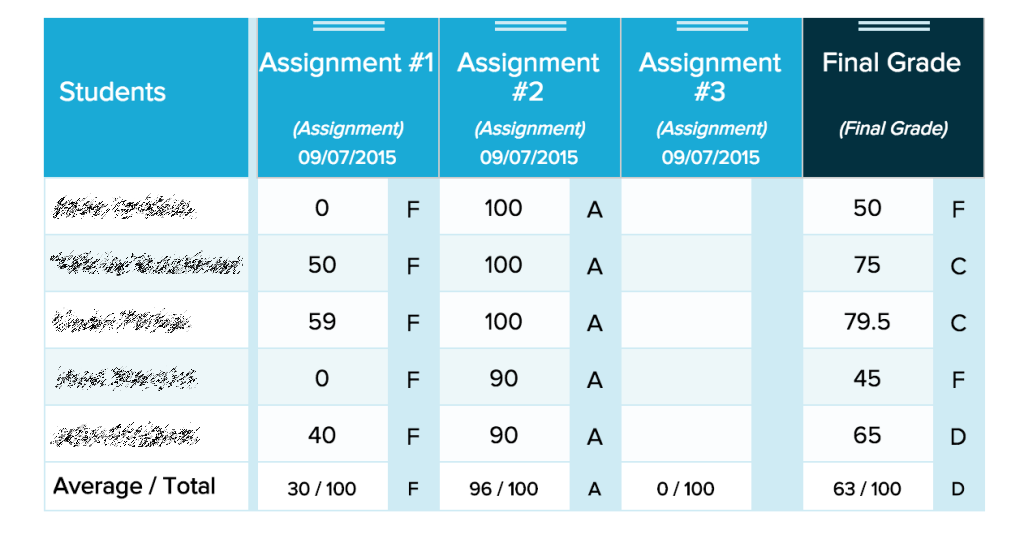The idea of Equal Interval is that an average of an A and an F should always yield a C (if they are equally set apart from one another). The average of an F and a C would always yield a D, and so on. When the interval between grade levels are the same, an F is no longer weighed so heavily (6 times more than any other grade level). And so you provide the student with an opportunity to dig themselves out of an F based on future effort.

There are many ways you can implement the Equal Interval philosophy within the QuickSchools Gradebook. Here we discuss a few options:

## Simple Conversion

### Convert raw marks to an equal interval scale.

The simplest approach is to mark each assignment using a 5-point scale:

In this fashion, when a student receives an F, regardless of the actual marks, you would assign ONE point. And if a student receives an A, you would assign 5 points. And when you calculate an average, you’re simply using the 5-point scale (NOTE: a 0-4 Scale will receive the same result):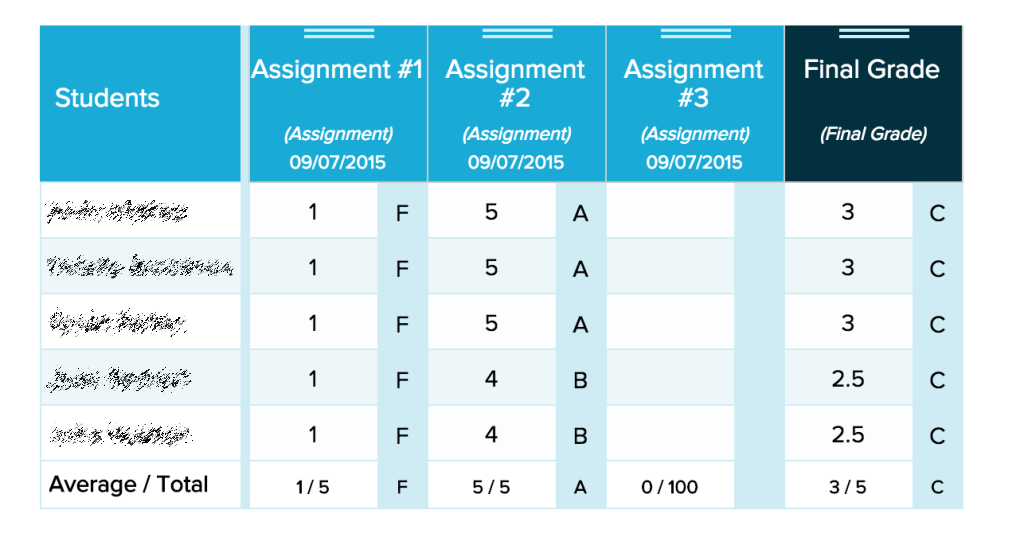Gradebook with Simple 5-point Equal Interval Scale

A side-effect of this method, is when you enter assignments in the Gradebook using a raw score that has not been converted to the 5-point scale. So for example, if you entered 100%, that would be an A, 80% would be a B, 60% would be a C, 40% would be a D, and 20% and below would be an F. The Grading Scale would also need to be modified slightly to fit this paradigm:

And the resulting Gradebook would look something like this: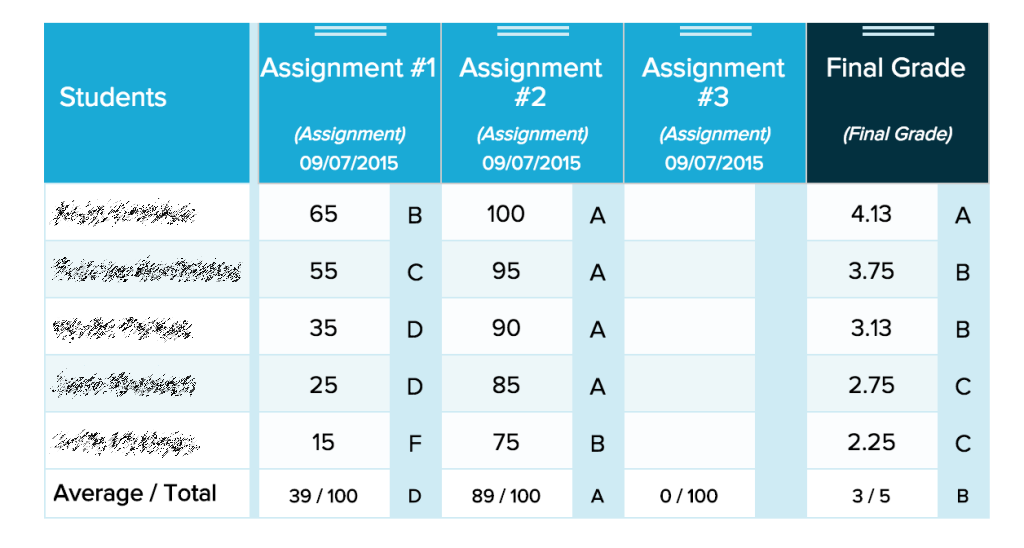Gradebook with 5-point Scale with Point Range

Unfortunately, the resulting calculation is a little counter-intuitive, since a student with say 50% mastery would receive a C. And a student would need to score a dismal 20% or less before failing. One way to address this, is to keep a conventional 100-point scale but introduce a point floor that limits the bottom 60% so that an F essentially has the same weight (or point range) as the other letter grades.

## Point Floor

### Implement a 50% point floor on a 100% scale.

This approach allows the user to enter a raw score. However, to implement the philosophy, we essentially start the scale at 50% (i.e. 90 - 100 is an A, 80 - 90 is a B, 70 - 80 is a C, 60 - 70 is a D, and 50 - 60 is an F). If someone scores below 50%, it’s simply regarded as 50%.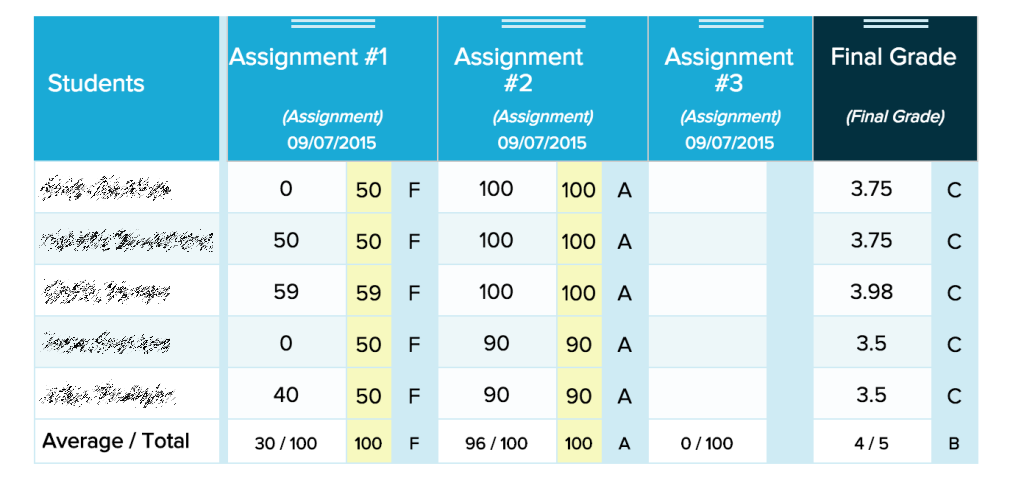In the example above, a student received 40% in an assignment, and that has been scaled up to 50%. Compare this with the "Conventional Gradebook Averaging" above, in this case, the average of an F and an A is always a C. You can still see the raw score for the assignment, but when calculating the final grade, the Gradebook will use the scaled up marks instead.

When implementing a Point Floor, you have to set the minimum percentage in two places, namely (1) in the Grading Scale as well as (2) in each individual column. For more details on this, check out our original post on Gradebook Calculations.

In this example, each assignment is never actually converted into a 5-point scale. Instead, the calculation is done on the raw score (which is adjusted for Equal Interval using the point floor). The final letter grade is then converted to the 5-point scale (ie. an A is 5 points, a B is 4 points, and so on).

The main issue with this method is that you can’t distinguish between a high performing F and a low performing F. And again, it seems counter-intuitive that a student can never receive below 50% for any given assignment.

## Hybrid Option

### Implement a Hybrid point-scale that automatically converts a raw score to the equal interval scale.

In this final method, users can enter a raw score, which then gets converted more accurately (than a point floor) to an equal interval scale. The converted 5-point score is then used in the final score calculation: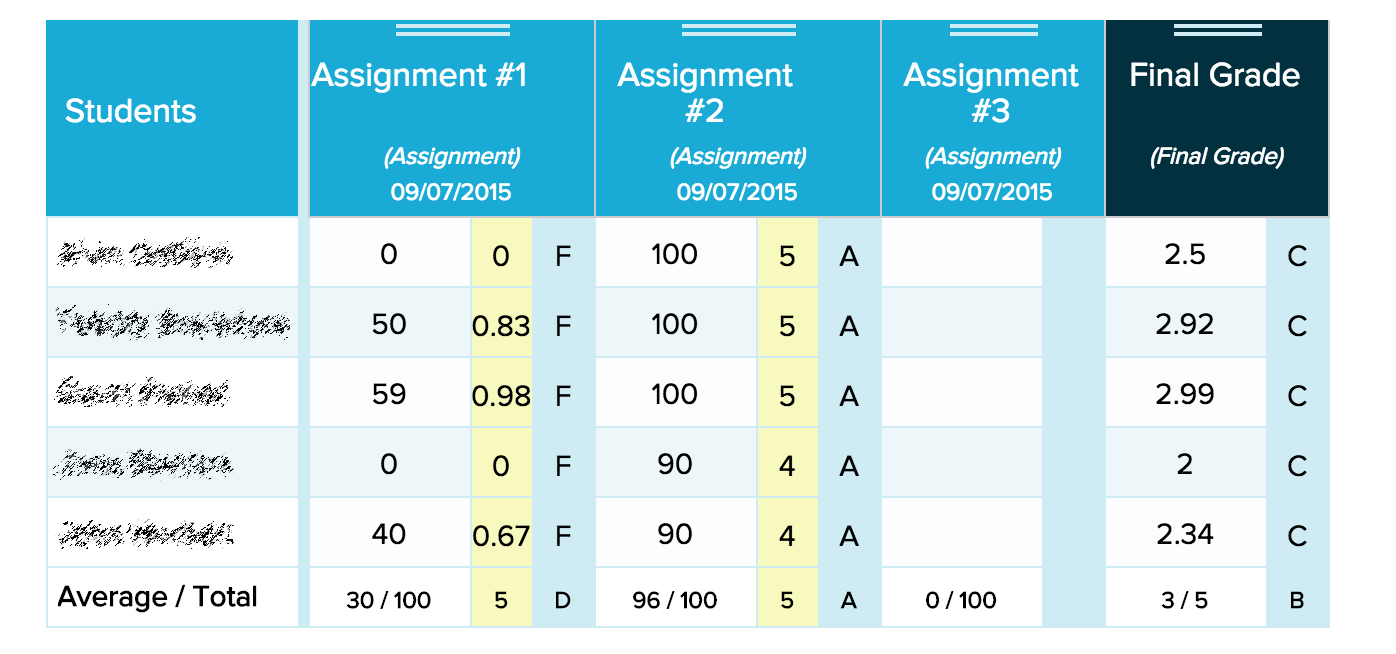Gradebook with Hybrid Equal Interval Scale

This method requires a more complicated scale that maps a raw score to a 5-point scale, and then to a letter grade:

This final method is more robust, but requires more setup. When setting up each individual column, instead of assigning a point floor, you have to still set the scale score. This is important if you also want to weight individual assignments more than others.

## Conclusion

Each method has it’s own merits, in terms of simplicity and accuracy. At the end of the day, the Gradebook is a tool for you to assess how the students are performing. If you have suggestions for improvement, please feel free to leave a comment, or come chat with us online.